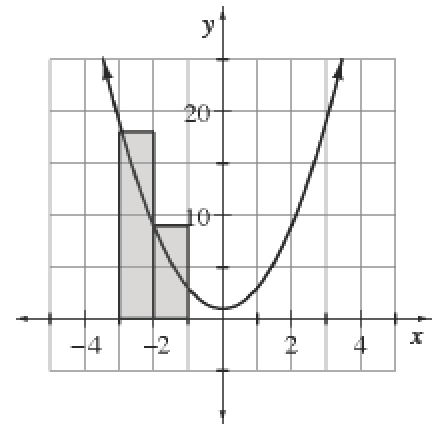### Home > APCALC > Chapter 1 > Lesson 1.2.3 > Problem1-58

1-58.

If for $f (x) = 2x^2 + 1$, estimate the area under the curve for $–3 ≤ x ≤ 3$ as follows.

1. Using six left endpoint rectangles. The first two rectangles are drawn for you.

Regarding the word ESTIMATE: You are not being asked to make a guess. You still need to compute. Of course, the area using rectangles is an approximation of the actual area under $f(x)$.

Sketch the remaining rectangle. Notice: The height of the LAST rectangle is evaluated at $x = 2$ (not $x = 3$).

Each rectangle has a base of $1$ and a height of $f(x)$. $\text{Area} = 1(f(−3) + f(−2) + f(−1) + f(0) + f(1) + f(2)) =$ ___________?

2. Using six right endpoint rectangles.

Notice: The height of the FIRST rectangle is evaluated at $x = −2$. The height of the LAST rectangle is evaluated at $x = 3$.

Each rectangle has a base of $1$ and a height of $f(x)$. $\text{Area} = 1( f(-2) + f(-1) + f(0) + f(1) + f(2) + f(3)) =$ __________?

3. Using six trapezoids. What do you notice? Does this always happen?

$A=\frac{1}{2}\left [ f(-3)+f(-2)+f(-1)+f(-1)+f(0)+f(0)+f(1)+f(1)+f(2)+f(2)+f(3) \right ]=\underline{ \ \ \ \ \ \ \ \ \ \ \ \ \ }$?

The trapezoidal sum is the AVERAGE of the left- and right-endpoint sums! Expain why this happens (algebraically and geometrically).Use the eTool below to view the graphs.
Click the link at right for the full version of the eTool: Calc 1-58 HW eTool